# ski-in / ski-out - Page 28

Viewing mode
689 results
• Slopes access :
• 50 -300m to the slopes
• Rooms :
• 3 rooms
• Total surface (sq.m) :
• 72 sq.m
•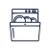•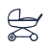•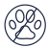• Slopes access :
• 50 -300m to the slopes
• Rooms :
• 4 rooms
• Total surface (sq.m) :
• 111 sq.m
• Slopes access :
• ski-in (-50 m)
• Rooms :
• Studio
• Studio + cabin(s)
• Total surface (sq.m) :
• 34 sq.m
•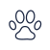•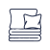•• Slopes access :
• ski-in (-50 m)
• Rooms :
• 2 rooms + cabin(s)
• 3 rooms
• Total surface (sq.m) :
• 53-68 sq.m
•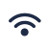•••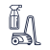•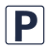•••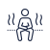•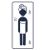• Slopes access :
• ski-in (-50 m)
• Total surface (sq.m) :
• 52 sq.m
••••• Slopes access :
• 50 -300m to the slopes
• Rooms :
• 4 rooms
• 4 rooms + cabin(s)
• Total surface (sq.m) :
• 103 sq.m
•••• Slopes access :
• ski-in (-50 m)
• Rooms :
• 2 rooms
• 2 rooms + cabin(s)
• Total surface (sq.m) :
• 51 sq.m
•••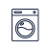••• Slopes access :
• ski-in (-50 m)
• Total surface (sq.m) :
• 41 sq.m
••••• Slopes access :
• 50 -300m to the slopes
• Rooms :
• 4 rooms
• Total surface (sq.m) :
• 59 sq.m
••••• Slopes access :
• ski-in (-50 m)
• Total surface (sq.m) :
• 32 sq.m
•••••• Slopes access :
• ski-in (-50 m)
• Rooms :
• Studio
• Total surface (sq.m) :
• 23 sq.m
••• Slopes access :
• 50 -300m to the slopes
• Rooms :
• 4 rooms
• Total surface (sq.m) :
• 85 sq.m
•••••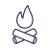••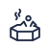•• Slopes access :
• 50 -300m to the slopes
• Rooms :
• 3 rooms
• Total surface (sq.m) :
• 45 sq.m
••••• Slopes access :
• ski-in (-50 m)
• Rooms :
• 7 rooms
• Total surface (sq.m) :
• 120 sq.m
•••• Slopes access :
• ski-in (-50 m)
• Total surface (sq.m) :
• 29 sq.m
•••••• Slopes access :
• 50 -300m to the slopes
• Rooms :
• 2 rooms
• Total surface (sq.m) :
• 30 sq.m
••• Slopes access :
• 50 -300m to the slopes
• Slopes access :
• 50 -300m to the slopes
• Rooms :
• 3 rooms
• 3 rooms + cabin(s)
• Total surface (sq.m) :
• 73 sq.m
•••• Slopes access :
• 50 -300m to the slopes
• Rooms :
• 2 rooms + cabin(s)
• Total surface (sq.m) :
• 25 sq.m
••••• Slopes access :
• 50 -300m to the slopes
• Rooms :
• 2 rooms
•••